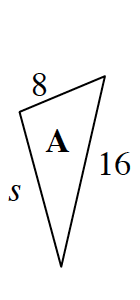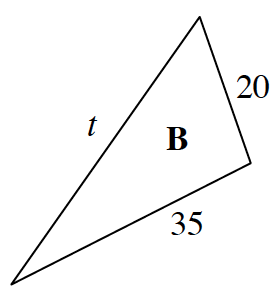### Home > ACC7 > Chapter 8 Unit 9 > Lesson CC3: 8.3.1 > Problem8-128

8-128.

The two triangles at right are similar shapes.

1. What is the scale factor between shape A and shape B?

Which corresponding sides in A and in B are both known?

What do you need to multiply $8$ by to get $20$?

$\frac{20}{8}=2.5$

2. Find the missing sides.

Identify which sides in A correspond to which sides in B.

Since $16$ corresponds to $t$ and $s$ corresponds to $35$, multiply $16$ by the scale factor to find $t$, and divide $35$ by the scale factor to find $s$.

3. If you wanted to make shape A smaller instead of bigger, what is a scale factor you could use?

The scale factor is what you multiply by to increase or decrease the size of a shape.

If a scale factor greater than one created a larger triangle, then a scale factor less than one must create a smaller triangle.
Note that a scale factor cannot be negative because a negative side length is nonexistent.